SSC CGL Previous Year Questions: Percentage - 1

# SSC CGL Previous Year Questions: Percentage - 1 - SSC CGL

Test Description

## 20 Questions MCQ Test SSC CGL (Tier - 1) - Previous Year Papers (Topic Wise) - SSC CGL Previous Year Questions: Percentage - 1

SSC CGL Previous Year Questions: Percentage - 1 for SSC CGL 2023 is part of SSC CGL (Tier - 1) - Previous Year Papers (Topic Wise) preparation. The SSC CGL Previous Year Questions: Percentage - 1 questions and answers have been prepared according to the SSC CGL exam syllabus.The SSC CGL Previous Year Questions: Percentage - 1 MCQs are made for SSC CGL 2023 Exam. Find important definitions, questions, notes, meanings, examples, exercises, MCQs and online tests for SSC CGL Previous Year Questions: Percentage - 1 below.
Solutions of SSC CGL Previous Year Questions: Percentage - 1 questions in English are available as part of our SSC CGL (Tier - 1) - Previous Year Papers (Topic Wise) for SSC CGL & SSC CGL Previous Year Questions: Percentage - 1 solutions in Hindi for SSC CGL (Tier - 1) - Previous Year Papers (Topic Wise) course. Download more important topics, notes, lectures and mock test series for SSC CGL Exam by signing up for free. Attempt SSC CGL Previous Year Questions: Percentage - 1 | 20 questions in 20 minutes | Mock test for SSC CGL preparation | Free important questions MCQ to study SSC CGL (Tier - 1) - Previous Year Papers (Topic Wise) for SSC CGL Exam | Download free PDF with solutions
 1 Crore+ students have signed up on EduRev. Have you?
SSC CGL Previous Year Questions: Percentage - 1 - Question 1

### Rahul’s salary is 40% less than Rakesh’s salary. Deepak’s salary is 80% more than Rahul’s salary. If Deepak’s salary is ₹  34560, then what is the salary of Rakesh?    (SSC MTS 2018)

Detailed Solution for SSC CGL Previous Year Questions: Percentage - 1 - Question 1

Let the salary of Rakesh be 100 unit
We have given Rahul's salary is 40%
Less than Rakesh's salary and Deepak's salary is 80% more than Rahul's salary.
So the ratio of the salaries are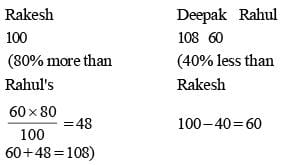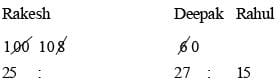we have Deepak's salary = ₹ 34560
27 unit → ₹ 34560
1 unit → ₹ 1280
Hence the salary of Rakesh is
= 1280 x 25 = ₹ 32000

SSC CGL Previous Year Questions: Percentage - 1 - Question 2

### The price of sugar is increased by 17%. A person wants to increase his expenditure by 5% only. By approximately what percent should be decrease his consumption?    (SSC CGL-2018)

Detailed Solution for SSC CGL Previous Year Questions: Percentage - 1 - Question 2

Let initial price of sugar was ₹ 100/kg
After increase price for 1kg sugar = ₹ 117
Increased Expenditure = ₹ 105
consumption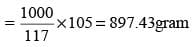∴ Decrease in consumption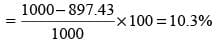SSC CGL Previous Year Questions: Percentage - 1 - Question 3

### An article is sold for ₹ 288 after successive discounts of 25% and x%. If the marked price of the article is ₹ 480, what is the value of x?    (SSC CGL-2018)

Detailed Solution for SSC CGL Previous Year Questions: Percentage - 1 - Question 3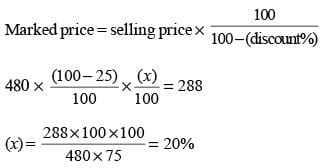SSC CGL Previous Year Questions: Percentage - 1 - Question 4

The price of sugar is increased by 20%. A person wants to increase his expenditure by 8% only. By what percent should he decrease his consumption?    (SSC CGL-2018)

Detailed Solution for SSC CGL Previous Year Questions: Percentage - 1 - Question 4

Let the original price of Sugar was ₹ 100/kg.
After increase, price of 1 kg Sugar = ₹ 120
Expenditure increases by 8%
So, new expenditure = 100 + 8 =  ₹ 108
Now, amount of Sugar bought in ₹ 108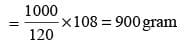Percent deduction in consumption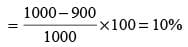SSC CGL Previous Year Questions: Percentage - 1 - Question 5

An article is sold for ₹ 528 after successive discounts of 20% and 12%. What is the marked price of the article?    (SSC CGL-2018)

Detailed Solution for SSC CGL Previous Year Questions: Percentage - 1 - Question 5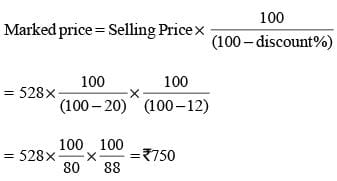SSC CGL Previous Year Questions: Percentage - 1 - Question 6

An article is subject to two successive discounts of 10% and 5% before being sold. If its marked price is ₹ 800, then its selling price is.    (SSC CHSL-2018)

Detailed Solution for SSC CGL Previous Year Questions: Percentage - 1 - Question 6

Selling price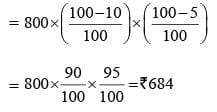SSC CGL Previous Year Questions: Percentage - 1 - Question 7

The price of sugar is decreased by 10%. By what percent can a person increase the consumption so that there is no change in the expenditure?    (SSC Sub. Ins. 2018 )

Detailed Solution for SSC CGL Previous Year Questions: Percentage - 1 - Question 7

Let price of 1 kg sugar is ₹100.
After decrease price of 1 kg sugar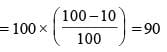Now, amount of sugar purchased in ₹ 100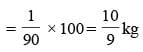Percentage increase in consumption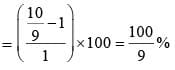SSC CGL Previous Year Questions: Percentage - 1 - Question 8

The successive discount of 25%, 20% and 10% is equivalent to a single discount of:    (SSC Sub. Ins.2018 )

Detailed Solution for SSC CGL Previous Year Questions: Percentage - 1 - Question 8

Amount after first discount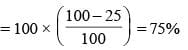Amount after second discount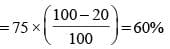Amount after third discount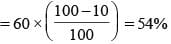∴ Equivalent discount = 100 – 54 = 46%

SSC CGL Previous Year Questions: Percentage - 1 - Question 9

In a class of 45 students, 40% are girls and the remaining are boys. The average marks of the girls is 64 and that of the boys is 60. What is the average marks of the whole class?    (SSC Sub. Ins. 2018 )

Detailed Solution for SSC CGL Previous Year Questions: Percentage - 1 - Question 9

Number of girls in the class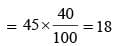Number of boys in the class = 45 – 18 = 27
Total marks of girls = 18 × 64 = 1152
Total marks of boys = 27 × 60 = 1620
Total marks of the class = 1152 + 1620 = 2772
Average marks of the class = 2772/45 = 61.6

SSC CGL Previous Year Questions: Percentage - 1 - Question 10

In an examination, 48% of candidates passed in science and 56% failed in mathematics. If 32% failed in both subjects, then what percent passed in both subjects?  (SSC Sub. Ins. 2018)

Detailed Solution for SSC CGL Previous Year Questions: Percentage - 1 - Question 10

Percent of students passed in mathematics
= 100 – 56 = 44%
Number of students passed in either science or math
= 100 – 32 = 68%
Number of students passed in both subjects
n(A ∩ B) = n(A) + n(B) – n(A ∪ B)
= 48% + 44% – 68%
= 24%

SSC CGL Previous Year Questions: Percentage - 1 - Question 11

The income of A is 24% more than the income of B. By what percent is the income of B less than the income of A?    (SSC Sub. Ins. 2018 )

Detailed Solution for SSC CGL Previous Year Questions: Percentage - 1 - Question 11

Let income of B is ₹ 100.
then income in A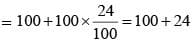Difference on income = 124 – 100 = 24.
Percentage difference in income of B w.r.t. A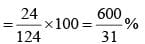SSC CGL Previous Year Questions: Percentage - 1 - Question 12

The price of table depreciates every year by 20%. If the value of the table after 2 years will be ₹ 32000, then what is the present price (in ₹) of the table?    (SSC Sub. Ins. 2017)

Detailed Solution for SSC CGL Previous Year Questions: Percentage - 1 - Question 12

Present price of table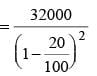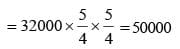SSC CGL Previous Year Questions: Percentage - 1 - Question 13

If 40% of a number is 290, then what is the number which is 20% more than the initial number?    (SSC Sub. Ins. 2017)

Detailed Solution for SSC CGL Previous Year Questions: Percentage - 1 - Question 13

Let original number = x
According to question,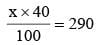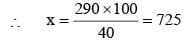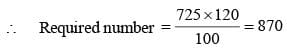SSC CGL Previous Year Questions: Percentage - 1 - Question 14

What will be the net discount (in percentage) after two successive discounts of 40% and 20%?    (SSC Sub. Ins. 2017)

Detailed Solution for SSC CGL Previous Year Questions: Percentage - 1 - Question 14

Required net discount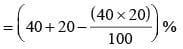= (60 – 8)% = 52%

SSC CGL Previous Year Questions: Percentage - 1 - Question 15

The population of a city increases at the rate of 5% per annum. If the present population of the city is 3,70,440. It population 3 years ago was:    (SSC MTS 2017)

Detailed Solution for SSC CGL Previous Year Questions: Percentage - 1 - Question 15

Present population = 370440
Rate = 5%
Time = 3 years
According to question,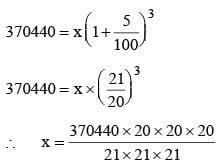= 320,000
∴ Population of city 3 years was = 320000.

SSC CGL Previous Year Questions: Percentage - 1 - Question 16

A person spends 25% of his annual income on house rent. 15% on education of children and 45% on other items. If he saves ₹ 14,400 annually, then the person's total income is:    (SSC MTS 2017)

Detailed Solution for SSC CGL Previous Year Questions: Percentage - 1 - Question 16

Tot al spend of  h is annual income
= (15% + 25% + 45%) = 85%
∴ Saves = (100 – 85)% = 15%
∴ 15% of annual income = 14400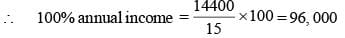∴ Total income = ₹ 96,000

SSC CGL Previous Year Questions: Percentage - 1 - Question 17

80 litre mixture of milk and water contains 10% milk. How much milk (in litres) must be added to make water percentage in the mixture as 80%?    (SSC CGL 2017)

Detailed Solution for SSC CGL Previous Year Questions: Percentage - 1 - Question 17

According to question,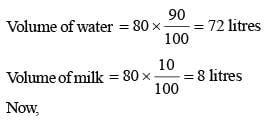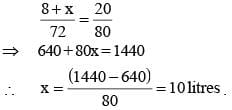SSC CGL Previous Year Questions: Percentage - 1 - Question 18

If A has got 20% more marks than B, then by what percent marks of B are less than the marks of A?    (SSC CGL 2017)

Detailed Solution for SSC CGL Previous Year Questions: Percentage - 1 - Question 18

Required percent of marks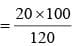= 16.66%

SSC CGL Previous Year Questions: Percentage - 1 - Question 19

After deducting 60% from a certain number and then deducting 15% from the remainder, 1428 is left. What was the initial number?    (SSC CGL 2017)

Detailed Solution for SSC CGL Previous Year Questions: Percentage - 1 - Question 19

Let initial number be x.
According to question,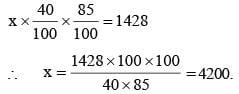SSC CGL Previous Year Questions: Percentage - 1 - Question 20

32% of a number exceeds 17% of the same number by 120. What is the value of the number?    (SSC CGL 2017)

Detailed Solution for SSC CGL Previous Year Questions: Percentage - 1 - Question 20

Required number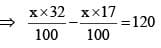## SSC CGL (Tier - 1) - Previous Year Papers (Topic Wise)

250 tests
Information about SSC CGL Previous Year Questions: Percentage - 1 Page
In this test you can find the Exam questions for SSC CGL Previous Year Questions: Percentage - 1 solved & explained in the simplest way possible. Besides giving Questions and answers for SSC CGL Previous Year Questions: Percentage - 1, EduRev gives you an ample number of Online tests for practice

250 tests